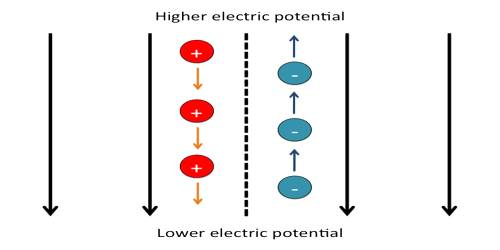# Point Measurement of Electric Potential

Point Measurement of Electric Potential

Definition: The amount of work done in bringing a unit positive charge from infinity to a point in an electric field is called electric potential at that point. It is denoted by V.

If W is the work done in bringing a positive charge q from infinity to a point in an electric field, the potential at that point, according to definition, is,

Work = Potential x charge, i. e., W = V x q

or, V = W/q = Work/Charge … … … … (1)

Since W and q in equation (1) are scalar quantities, so V is also a scalar quantity. But potential V has a sign. The potential at a point can be positive or negative. If the electric field is due to positive charge, i.e., if the field is positive, then in order to bring a unit positive from infinity work is to be done against the repulsive force of the similar charges. So potential due to a positive charge is positive. But, if the field is due to negative charge, then no work is to be done by external force to bring a unit positive charge from infinity. The attractive force will act on this positive and negative charges. So, potential due to a negative charge is negative.

Unit: In S. I. method, the unit of energy is Joule and the unit of charge is Coulomb.

So, the unit of electric potential,

V = Joule / Coulomb

[Joule / Coulomb] unit of electric potential is called volt.

1 volt potential: If 1 Joule of work is done in bringing 1 Coulomb of positive charge from infinity to a point in the electric field then the potential at that point is called 1 volt.